# CostVolumeProfit Relationships Chapter 5 Power Point Authors Susan

• Slides: 97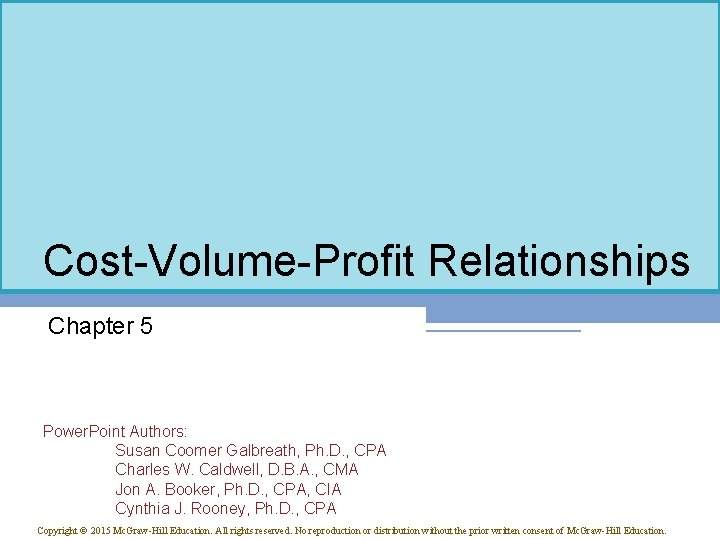Cost-Volume-Profit Relationships Chapter 5 Power. Point Authors: Susan Coomer Galbreath, Ph. D. , CPA Charles W. Caldwell, D. B. A. , CMA Jon A. Booker, Ph. D. , CPA, CIA Cynthia J. Rooney, Ph. D. , CPA Copyright © 2015 Mc. Graw-Hill Education. All rights reserved. No reproduction or distribution without the prior written consent of Mc. Graw-Hill Education.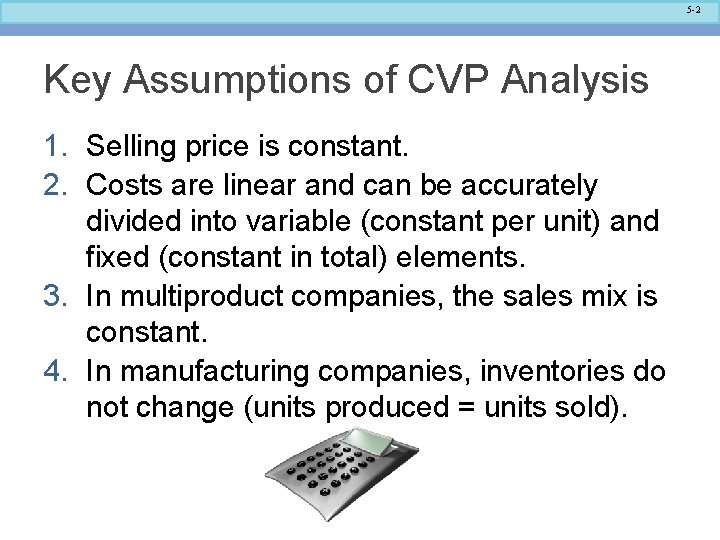5 -2 Key Assumptions of CVP Analysis 1. Selling price is constant. 2. Costs are linear and can be accurately divided into variable (constant per unit) and fixed (constant in total) elements. 3. In multiproduct companies, the sales mix is constant. 4. In manufacturing companies, inventories do not change (units produced = units sold).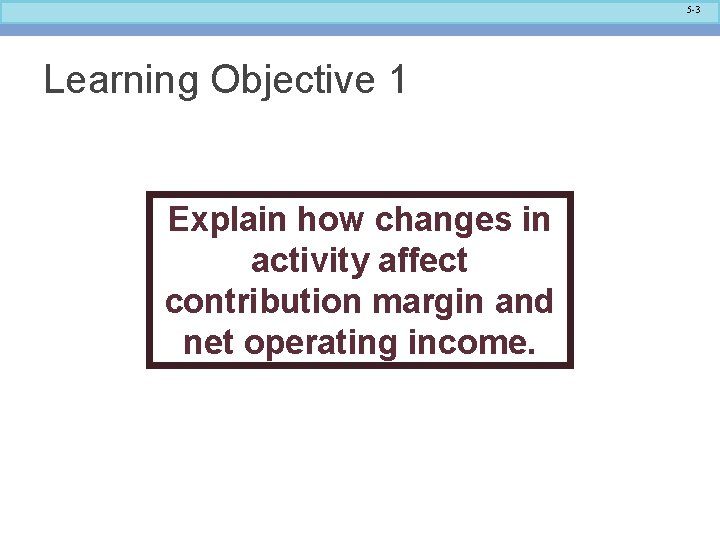5 -3 Learning Objective 1 Explain how changes in activity affect contribution margin and net operating income.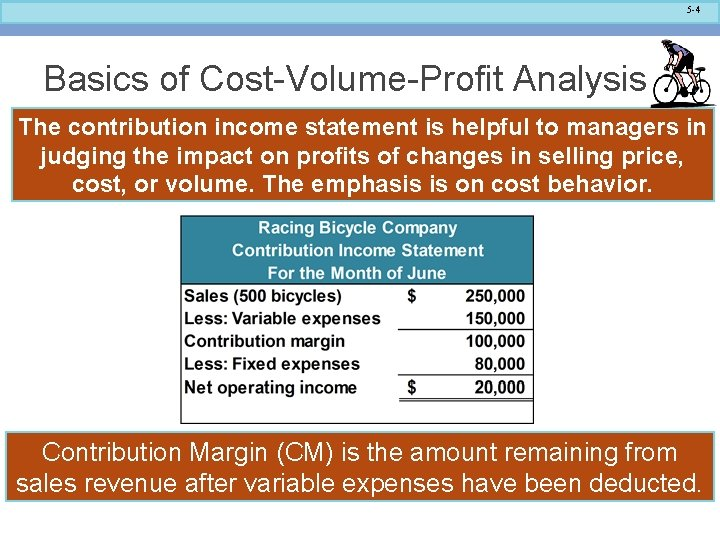5 -4 Basics of Cost-Volume-Profit Analysis The contribution income statement is helpful to managers in judging the impact on profits of changes in selling price, cost, or volume. The emphasis is on cost behavior. Contribution Margin (CM) is the amount remaining from sales revenue after variable expenses have been deducted.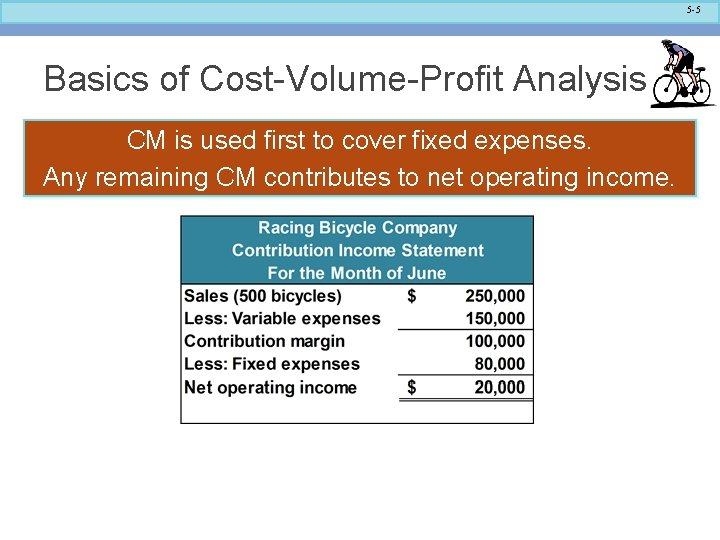5 -5 Basics of Cost-Volume-Profit Analysis CM is used first to cover fixed expenses. Any remaining CM contributes to net operating income.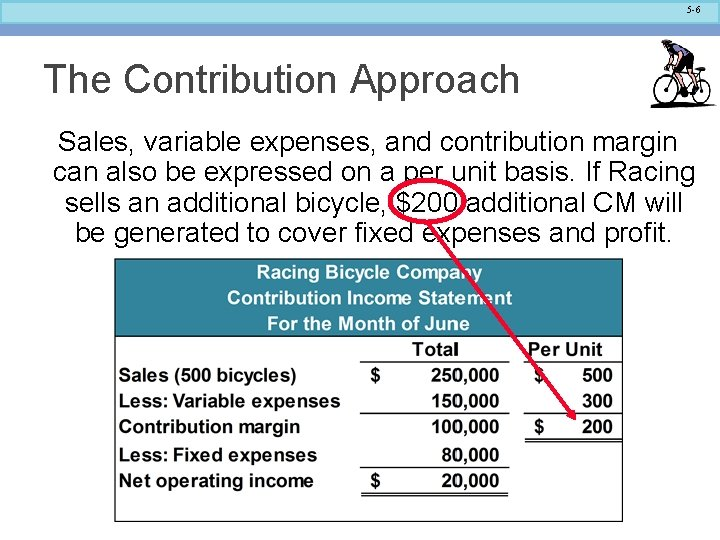5 -6 The Contribution Approach Sales, variable expenses, and contribution margin can also be expressed on a per unit basis. If Racing sells an additional bicycle, \$200 additional CM will be generated to cover fixed expenses and profit.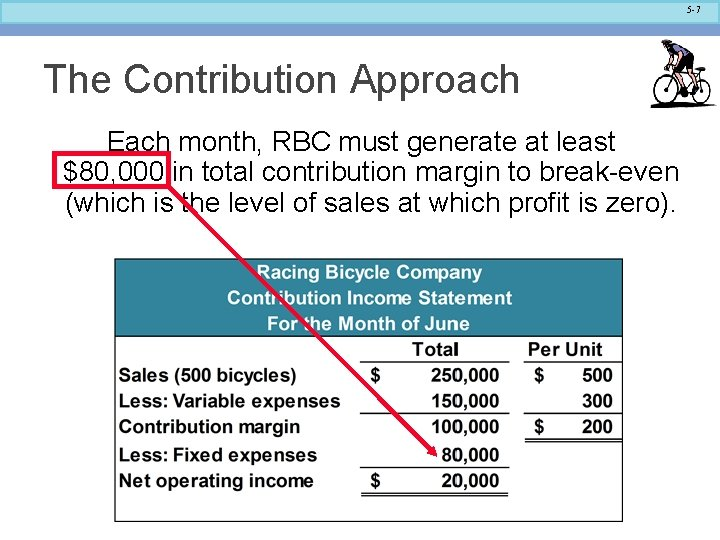5 -7 The Contribution Approach Each month, RBC must generate at least \$80, 000 in total contribution margin to break-even (which is the level of sales at which profit is zero).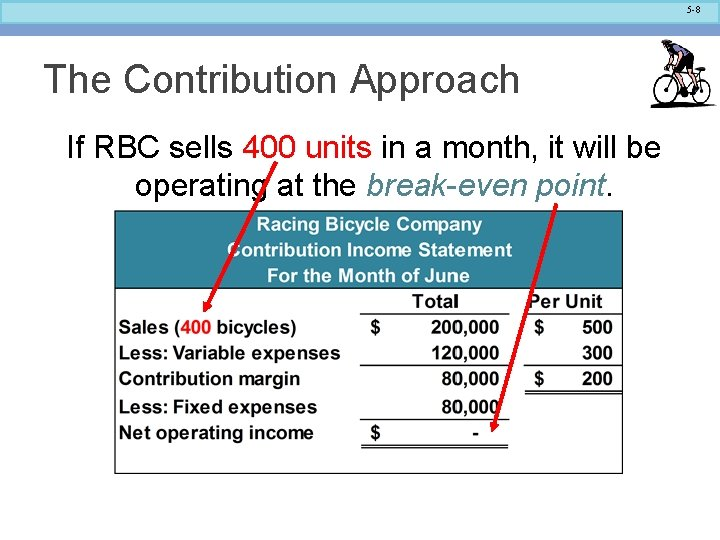5 -8 The Contribution Approach If RBC sells 400 units in a month, it will be operating at the break-even point.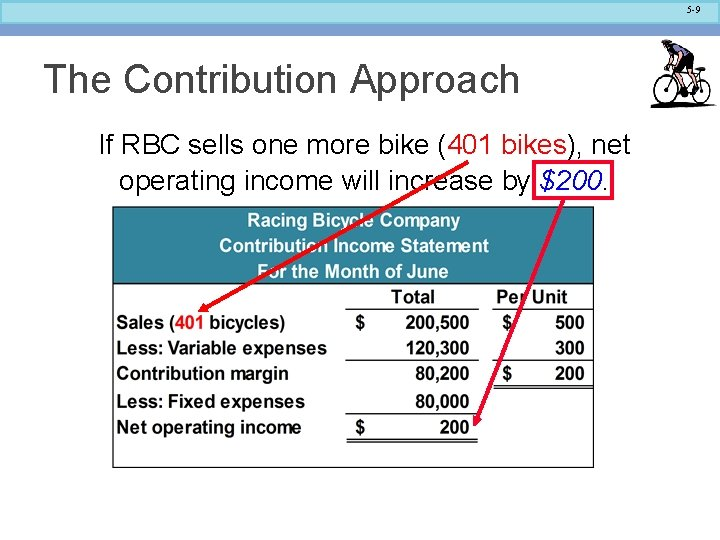5 -9 The Contribution Approach If RBC sells one more bike (401 bikes), net operating income will increase by \$200.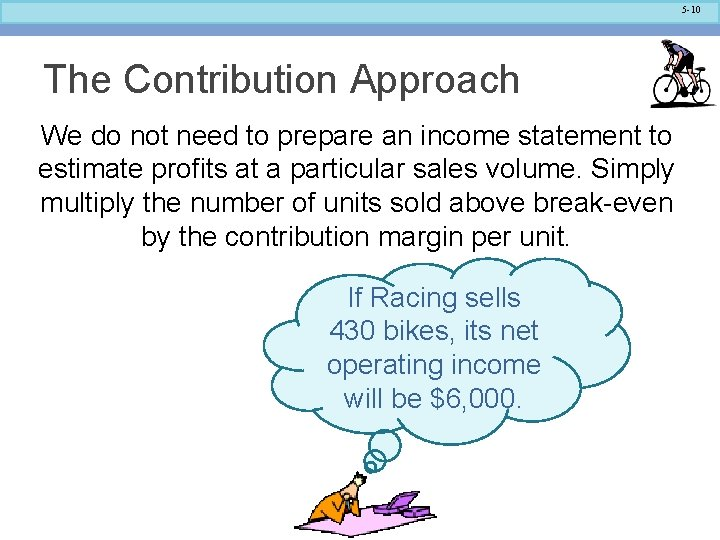5 -10 The Contribution Approach We do not need to prepare an income statement to estimate profits at a particular sales volume. Simply multiply the number of units sold above break-even by the contribution margin per unit. If Racing sells 430 bikes, its net operating income will be \$6, 000.5 -11 CVP Relationships in Equation Form The contribution format income statement can be expressed in the following equation: Profit = (Sales – Variable expenses) – Fixed expenses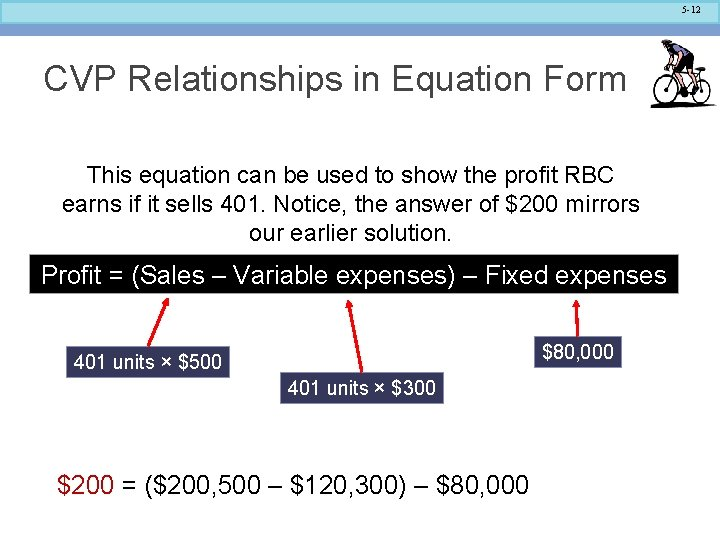5 -12 CVP Relationships in Equation Form This equation can be used to show the profit RBC earns if it sells 401. Notice, the answer of \$200 mirrors our earlier solution. Profit = (Sales – Variable expenses) – Fixed expenses \$80, 000 401 units × \$500 401 units × \$300 Profit \$200 = (\$200, 500 – \$120, 300) Variable expenses) – \$80, 000 Fixed expenses – Fixed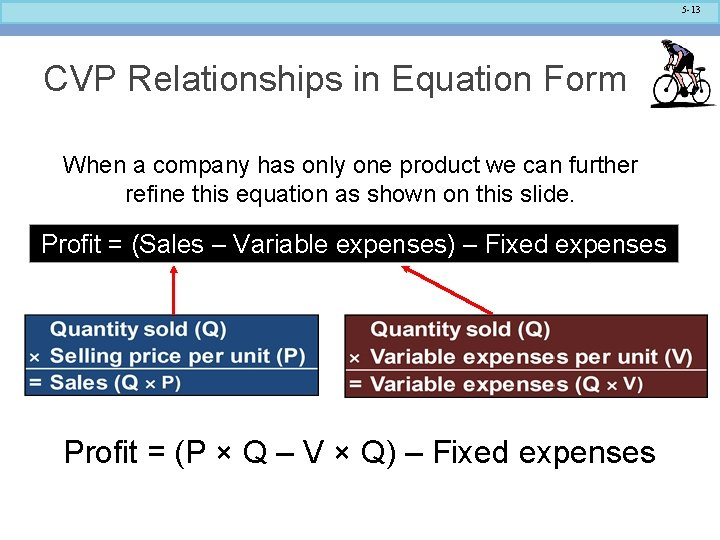5 -13 CVP Relationships in Equation Form When a company has only one product we can further refine this equation as shown on this slide. Profit = (Sales – Variable expenses) – Fixed expenses Profit = (P × Q – V × Q) – Fixed expenses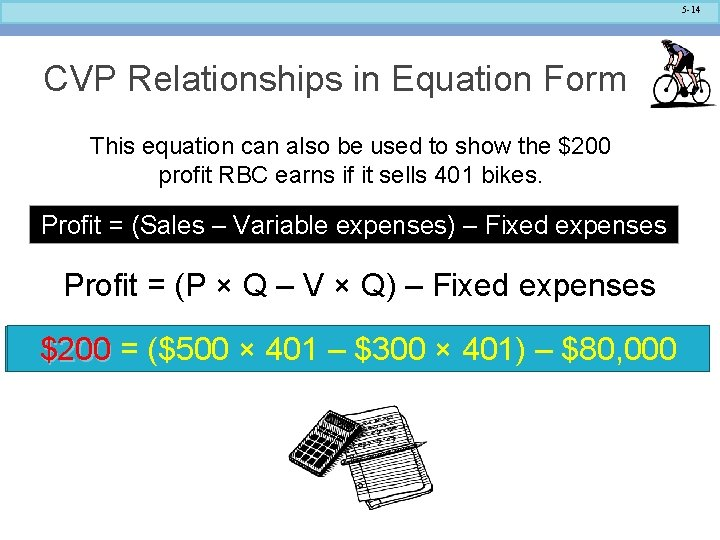5 -14 CVP Relationships in Equation Form This equation can also be used to show the \$200 profit RBC earns if it sells 401 bikes. Profit = (Sales – Variable expenses) – Fixed expenses Profit = (P × Q – V × Q) – Fixed expenses \$200 = (\$500 × 401 – \$300 × 401) – \$80, 000 Profit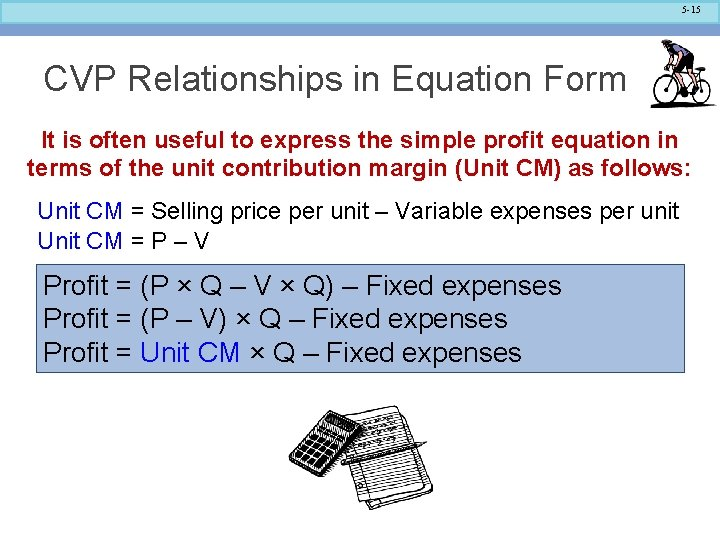5 -15 CVP Relationships in Equation Form It is often useful to express the simple profit equation in terms of the unit contribution margin (Unit CM) as follows: Unit CM = Selling price per unit – Variable expenses per unit Unit CM = P – V Profit = (P × Q – V × Q) – Fixed expenses Profit = (P – V) × Q – Fixed expenses Profit = Unit CM × Q – Fixed expenses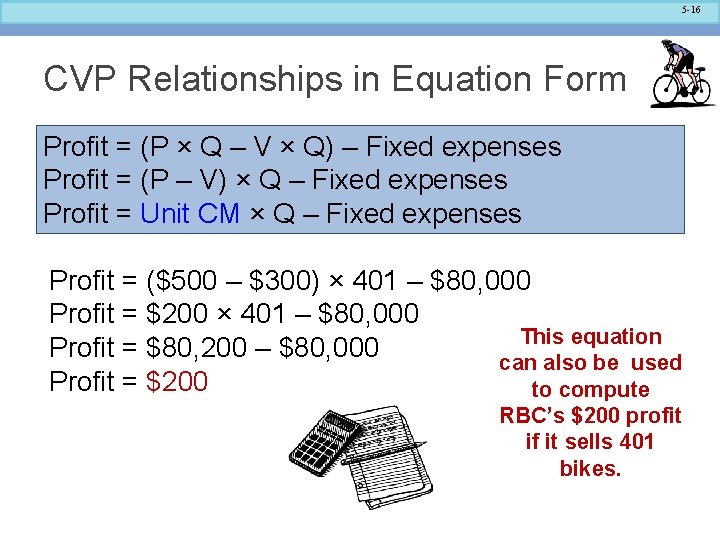5 -16 CVP Relationships in Equation Form Profit = (P × Q – V × Q) – Fixed expenses Profit = (P – V) × Q – Fixed expenses Profit = Unit CM × Q – Fixed expenses Profit = (\$500 – \$300) × 401 – \$80, 000 Profit = \$200 × 401 – \$80, 000 This equation Profit = \$80, 200 – \$80, 000 can also be used Profit = \$200 to compute RBC’s \$200 profit if it sells 401 bikes.5 -17 Learning Objective 2 Prepare and interpret a cost-volume-profit (CVP) graph and a profit graph.5 -18 CVP Relationships in Graphic Form The relationships among revenue, cost, profit, and volume can be expressed graphically by preparing a CVP graph. Racing Bicycle developed contribution margin income statements at 0, 200, 400, and 600 units sold. We will use this information to prepare the CVP graph.5 -19 Preparing the CVP Graph \$350, 000 \$300, 000 Dollars \$250, 000 \$200, 000 \$150, 000 In a CVP graph, unit volume is usually represented on the horizontal (X) axis and dollars on the vertical (Y) axis. \$100, 000 \$50, 000 \$0 0 100 200 300 Units 400 500 6005 -20 Preparing the CVP Graph Draw a line parallel to the volume axis to represent total fixed expenses. \$350, 000 \$300, 000 Dollars \$250, 000 \$200, 000 Fixed expenses \$150, 000 \$100, 000 \$50, 000 \$0 0 100 200 300 400 Units 500 6005 -21 Preparing the CVP Graph Choose some sales volume, say 400 units, and plot the point representing \$300, 000 total expenses (fixed and variable). Draw a line through the data point back to where the fixed expenses line intersects the dollar axis. \$350, 000 Dollars \$250, 000 \$200, 000 Total expenses Fixed expenses \$150, 000 \$100, 000 \$50, 000 \$0 0 100 200 300 400 Units 500 600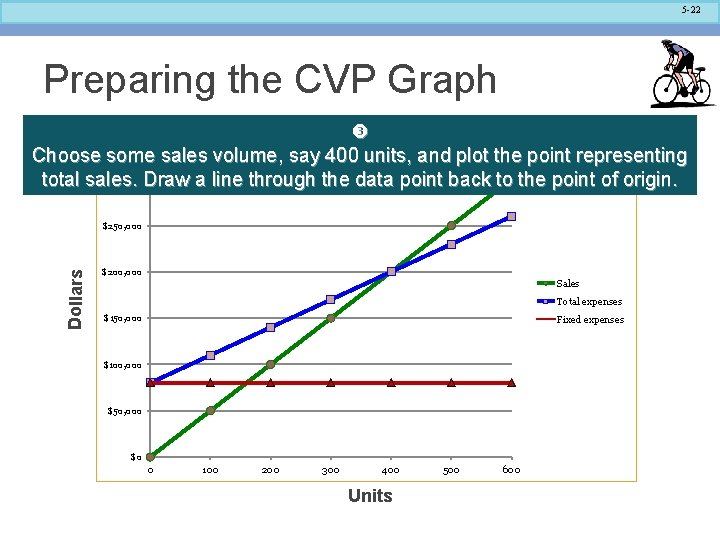5 -22 Preparing the CVP Graph Choose some sales volume, say 400 units, and plot the point representing \$300, 000 Draw a line through the data point back to the point of origin. total sales. \$350, 000 Dollars \$250, 000 \$200, 000 Sales Total expenses \$150, 000 Fixed expenses \$100, 000 \$50, 000 \$0 0 100 200 300 400 Units 500 600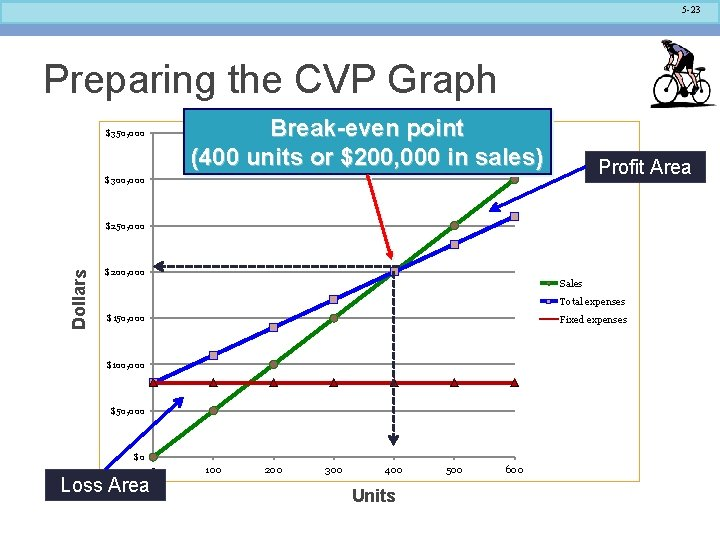5 -23 Preparing the CVP Graph Break-even point (400 units or \$200, 000 in sales) \$350, 000 Profit Area \$300, 000 Dollars \$250, 000 \$200, 000 Sales Total expenses \$150, 000 Fixed expenses \$100, 000 \$50, 000 \$0 Loss Area 0 100 200 300 400 Units 500 6005 -24 Preparing the CVP Graph Profit = Unit CM × Q – Fixed Costs An even simpler form of the CVP graph is called the profit graph.5 -25 Preparing the CVP Graph Break-even point, where profit is zero, is 400 units sold.5 -26 Learning Objective 3 Use the contribution margin ration (CM ratio) to compute changes in contribution margin and net operating income resulting from changes in sales volume.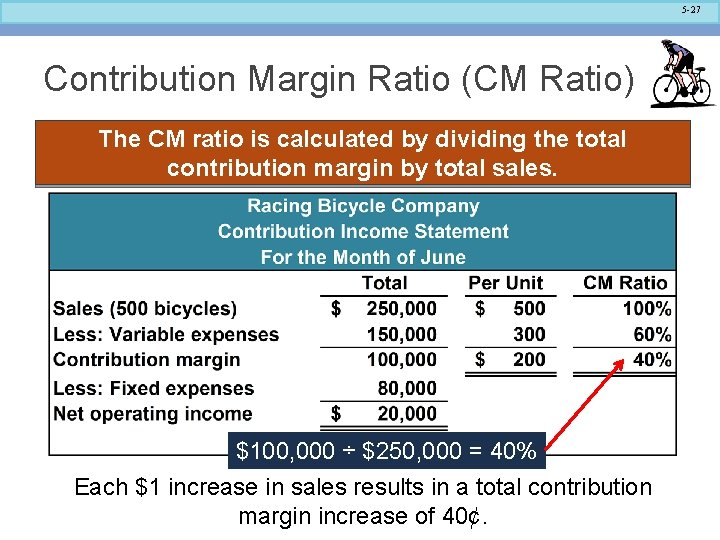5 -27 Contribution Margin Ratio (CM Ratio) The CM ratio is calculated by dividing the total contribution margin by total sales. \$100, 000 ÷ \$250, 000 = 40% Each \$1 increase in sales results in a total contribution margin increase of 40¢.5 -28 Contribution Margin Ratio (CM Ratio) The contribution margin ratio at Racing Bicycle is: CM per unit = CM Ratio = SP per unit \$200 \$500 = 40% The CM ratio can also be calculated by dividing the contribution margin per unit by the selling price per unit.5 -29 Contribution Margin Ratio (CM Ratio) If Racing Bicycle increases sales from 400 to 500 bikes (\$50, 000), contribution margin will increase by \$20, 000 (\$50, 000 × 40%). Here is the proof: A \$50, 000 increase in sales revenue results in a \$20, 000 increase in CM (\$50, 000 × 40% = \$20, 000).5 -30 Quick Check Coffee Klatch is an espresso stand in a downtown office building. The average selling price of a cup of coffee is \$1. 49 and the average variable expense per cup is \$0. 36. The average fixed expense per month is \$1, 300. An average of 2, 100 cups are sold each month. What is the CM Ratio for Coffee Klatch? a. 1. 319 b. 0. 758 c. 0. 242 d. 4. 1395 -31 Quick Check Coffee Klatch is an espresso stand in a downtown office building. The average selling price of a cup of coffee is \$1. 49 and the average variable expense per cup is \$0. 36. The average fixed expense per month is \$1, 300. An average of 2, 100 cups are sold each month. What is the CM Ratio for Coffee Klatch? a. 1. 319 Unit contribution margin CM Ratio = b. 0. 758 Unit selling price c. 0. 242 (\$1. 49 - \$0. 36) = d. 4. 139 \$1. 49 = \$1. 13 = 0. 758 \$1. 49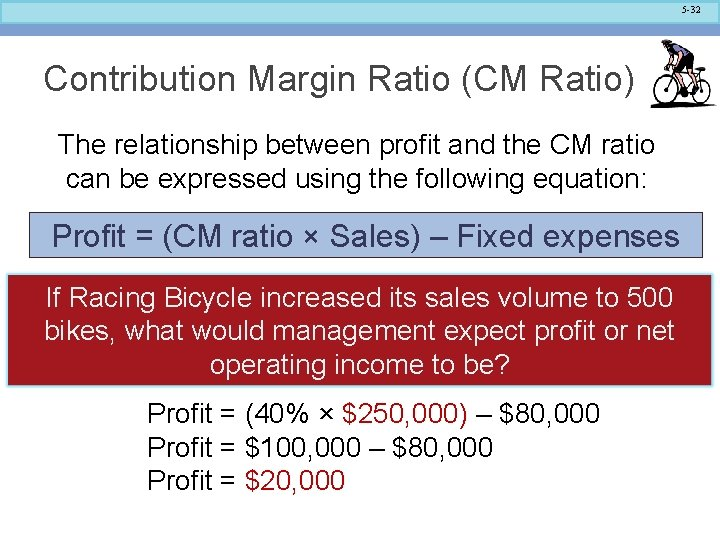5 -32 Contribution Margin Ratio (CM Ratio) The relationship between profit and the CM ratio can be expressed using the following equation: Profit = (CM ratio × Sales) – Fixed expenses If Racing Bicycle increased its sales volume to 500 bikes, what would management expect profit or net operating income to be? Profit = (40% × \$250, 000) – \$80, 000 Profit = \$100, 000 – \$80, 000 Profit = \$20, 0005 -33 Learning Objective 4 Show the effects on net operating income of changes in variable costs, fixed costs, selling price, and volume.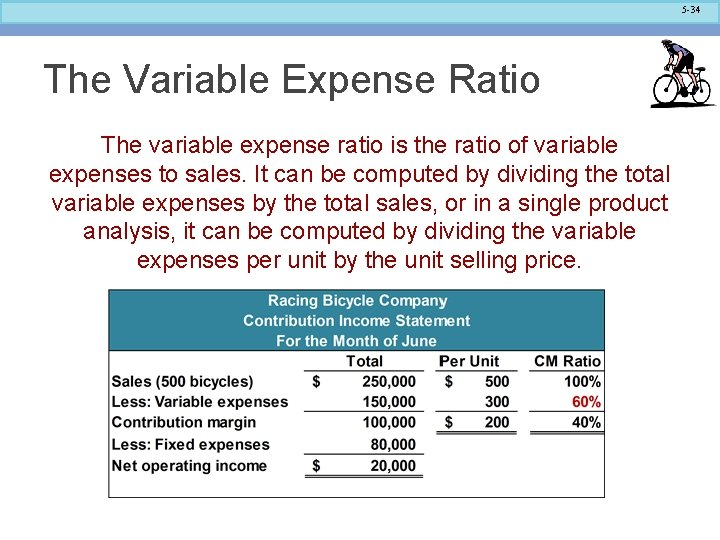5 -34 The Variable Expense Ratio The variable expense ratio is the ratio of variable expenses to sales. It can be computed by dividing the total variable expenses by the total sales, or in a single product analysis, it can be computed by dividing the variable expenses per unit by the unit selling price.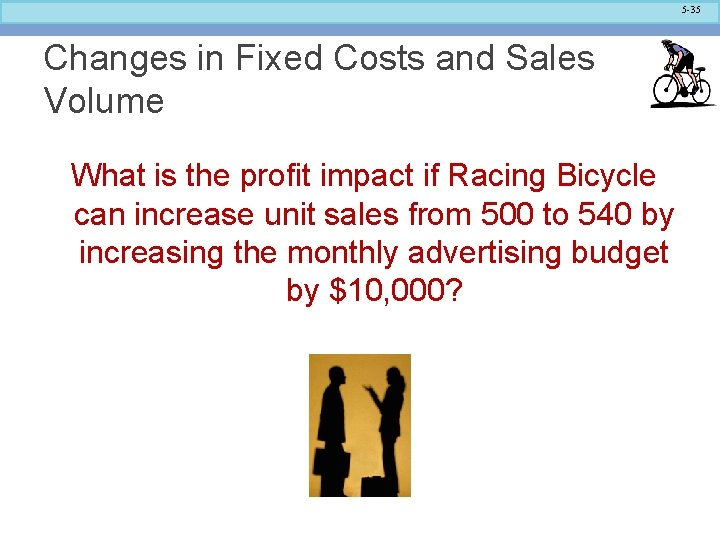5 -35 Changes in Fixed Costs and Sales Volume What is the profit impact if Racing Bicycle can increase unit sales from 500 to 540 by increasing the monthly advertising budget by \$10, 000?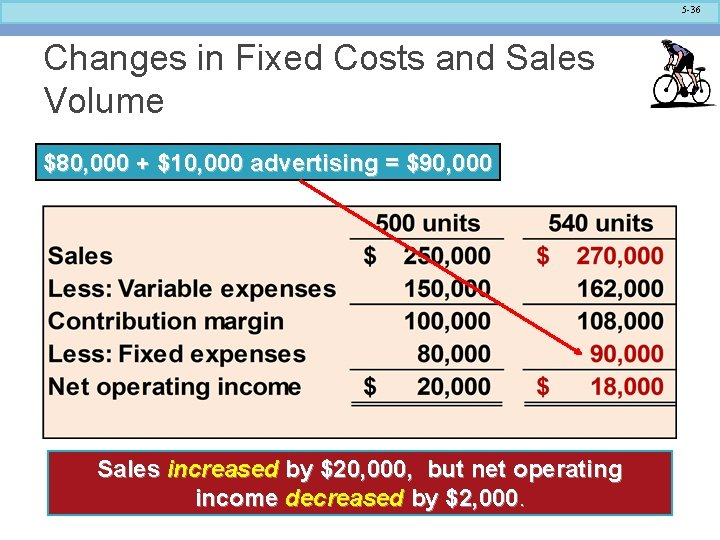5 -36 Changes in Fixed Costs and Sales Volume \$80, 000 + \$10, 000 advertising = \$90, 000 Sales increased by \$20, 000, but net operating income decreased by \$2, 000.5 -37 Changes in Fixed Costs and Sales Volume A shortcut solution using incremental analysis5 -38 Change in Variable Costs and Sales Volume What is the profit impact if Racing Bicycle can use higher quality raw materials, thus increasing variable costs per unit by \$10, to generate an increase in unit sales from 500 to 580?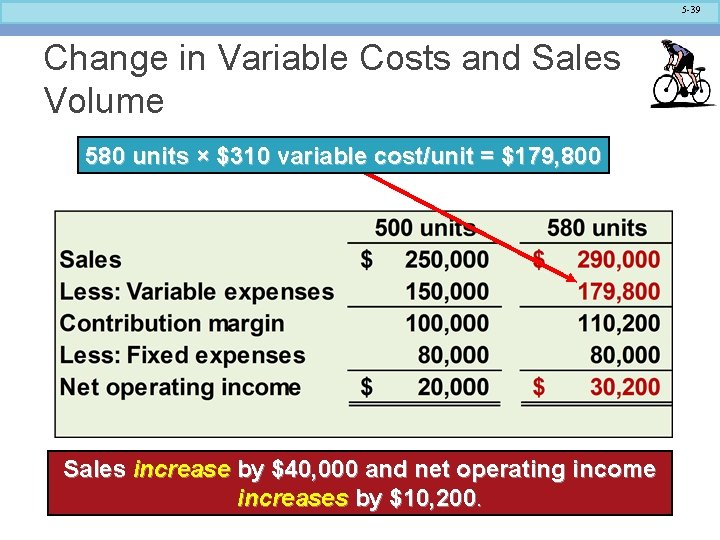5 -39 Change in Variable Costs and Sales Volume 580 units × \$310 variable cost/unit = \$179, 800 Sales increase by \$40, 000 and net operating income increases by \$10, 200.5 -40 Change in Fixed Cost, Sales Price, and Volume What is the profit impact if RBC: (1) cuts its selling price \$20 per unit, (2) increases its advertising budget by \$15, 000 per month, and (3) increases sales from 500 to 650 units per month?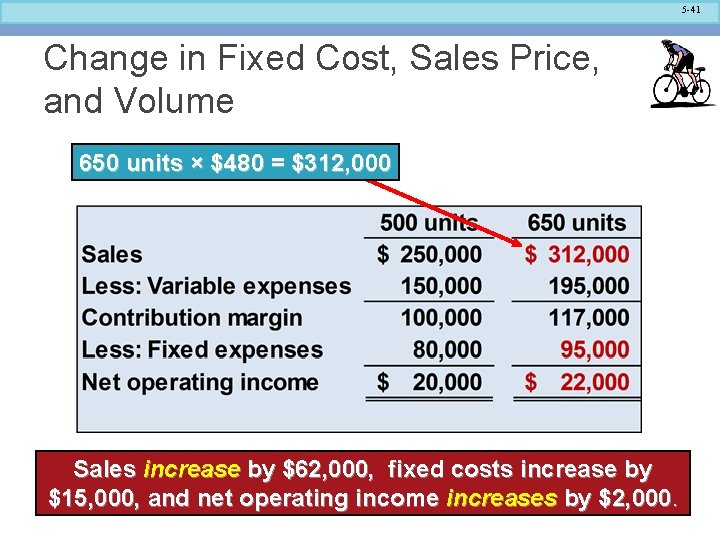5 -41 Change in Fixed Cost, Sales Price, and Volume 650 units × \$480 = \$312, 000 Sales increase by \$62, 000, fixed costs increase by \$15, 000, and net operating income increases by \$2, 000.5 -42 Change in Variable Cost, Fixed Cost, and Sales Volume What is the profit impact if RBC: (1) pays a \$15 sales commission per bike sold instead of paying salespersons flat salaries that currently total \$6, 000 per month, and (2) increases unit sales from 500 to 575 bikes?5 -43 Change in Variable Cost, Fixed Cost, and Sales Volume 575 units × \$315 = \$181, 125 Sales increase by \$37, 500, fixed expenses decrease by \$6, 000, and net operating income increases by \$12, 375.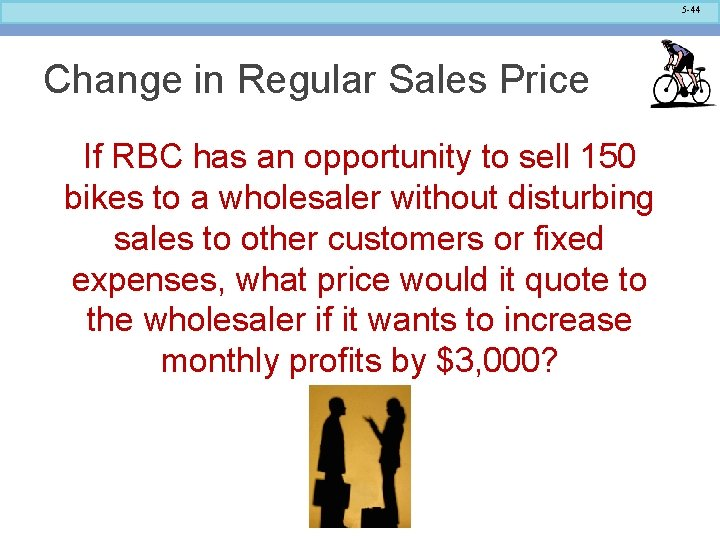5 -44 Change in Regular Sales Price If RBC has an opportunity to sell 150 bikes to a wholesaler without disturbing sales to other customers or fixed expenses, what price would it quote to the wholesaler if it wants to increase monthly profits by \$3, 000?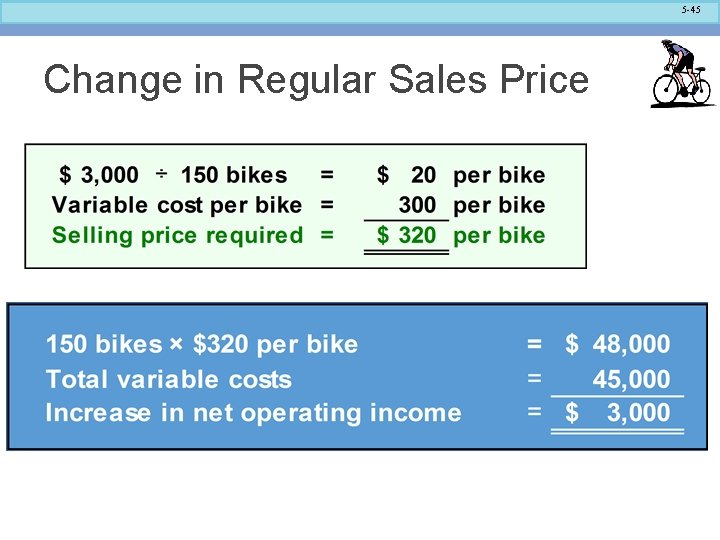5 -45 Change in Regular Sales Price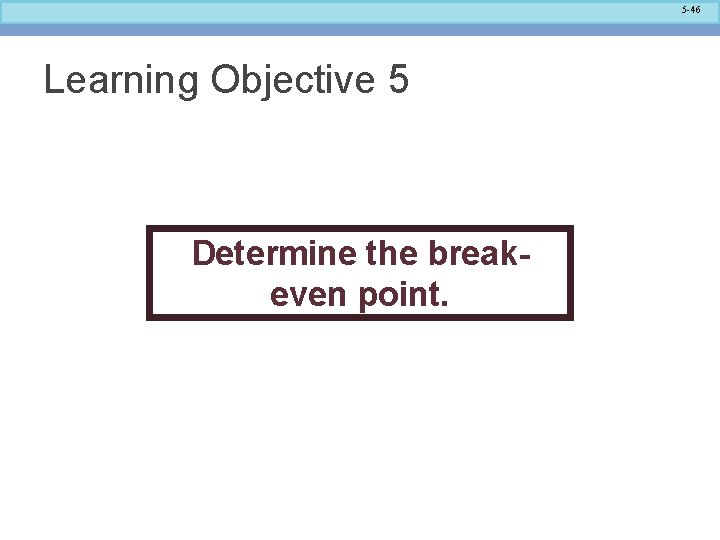5 -46 Learning Objective 5 Determine the breakeven point.5 -47 Break-even Analysis The equation and formula methods can be used to determine the unit sales and dollar sales needed to achieve a target profit of zero. Let’s use the RBC information to complete the break-even analysis.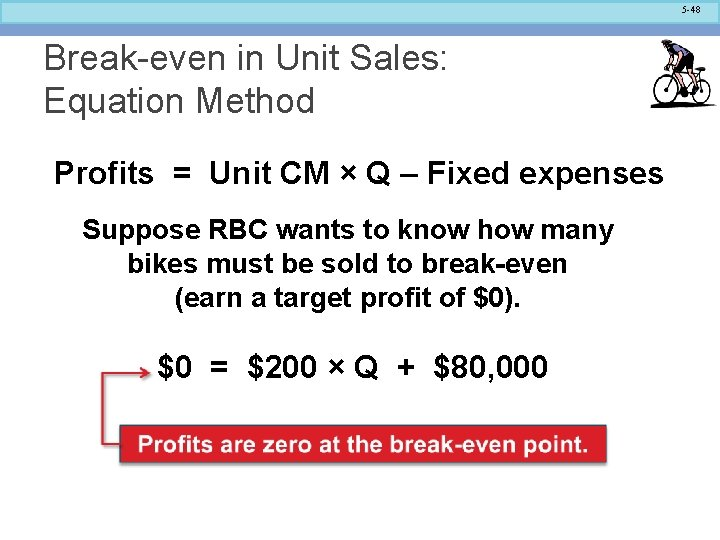5 -48 Break-even in Unit Sales: Equation Method Profits = Unit CM × Q – Fixed expenses Suppose RBC wants to know how many bikes must be sold to break-even (earn a target profit of \$0). \$0 = \$200 × Q + \$80, 000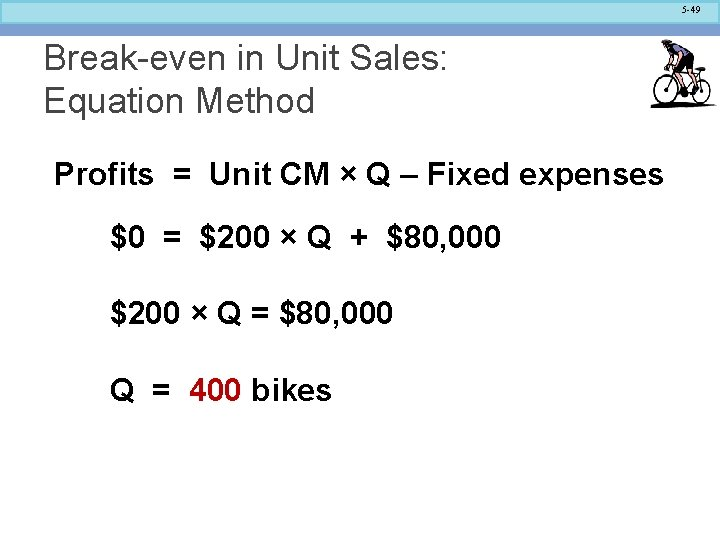5 -49 Break-even in Unit Sales: Equation Method Profits = Unit CM × Q – Fixed expenses \$0 = \$200 × Q + \$80, 000 \$200 × Q = \$80, 000 Q = 400 bikes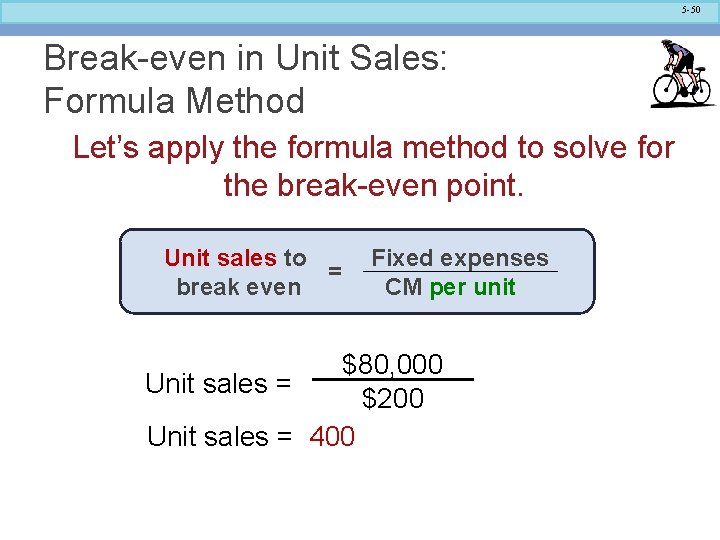5 -50 Break-even in Unit Sales: Formula Method Let’s apply the formula method to solve for the break-even point. Unit sales to = break even Fixed expenses CM per unit \$80, 000 Unit sales = \$200 Unit sales = 4005 -51 Break-even in Dollar Sales: Equation Method Suppose Racing Bicycle wants to compute the sales dollars required to break-even (earn a target profit of \$0). Let’s use the equation method to solve this problem. Profit = CM ratio × Sales – Fixed expenses Solve for the unknown “Sales. ”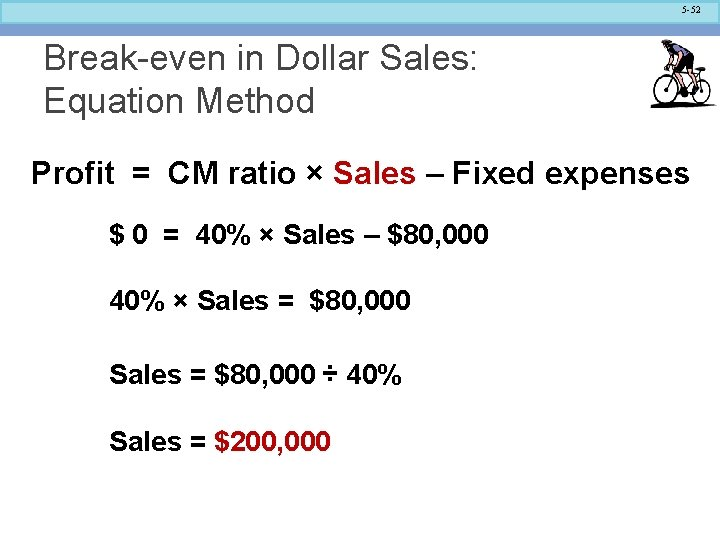5 -52 Break-even in Dollar Sales: Equation Method Profit = CM ratio × Sales – Fixed expenses \$ 0 = 40% × Sales – \$80, 000 40% × Sales = \$80, 000 ÷ 40% Sales = \$200, 000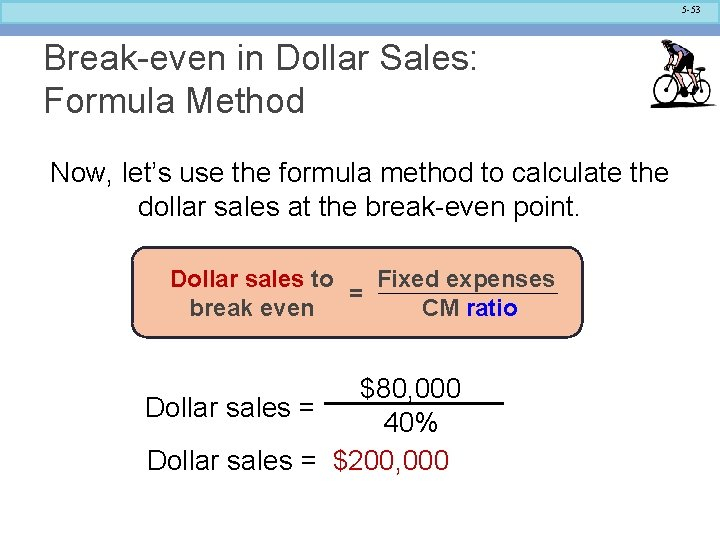5 -53 Break-even in Dollar Sales: Formula Method Now, let’s use the formula method to calculate the dollar sales at the break-even point. Dollar sales to Fixed expenses = break even CM ratio \$80, 000 Dollar sales = 40% Dollar sales = \$200, 0005 -54 Quick Check Coffee Klatch is an espresso stand in a downtown office building. The average selling price of a cup of coffee is \$1. 49 and the average variable expense per cup is \$0. 36. The average fixed expense per month is \$1, 300. An average of 2, 100 cups are sold each month. What is the break-even sales dollars? a. \$1, 300 b. \$1, 715 c. \$1, 788 d. \$3, 129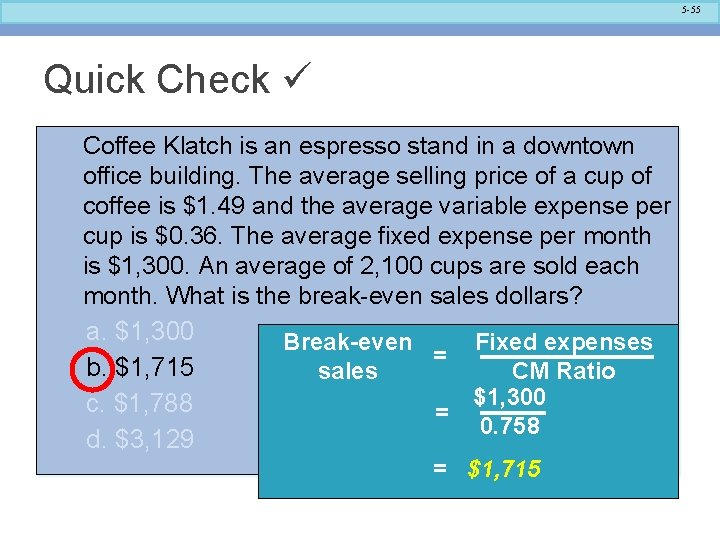5 -55 Quick Check Coffee Klatch is an espresso stand in a downtown office building. The average selling price of a cup of coffee is \$1. 49 and the average variable expense per cup is \$0. 36. The average fixed expense per month is \$1, 300. An average of 2, 100 cups are sold each month. What is the break-even sales dollars? a. \$1, 300 Fixed expenses Break-even = b. \$1, 715 CM Ratio sales \$1, 300 c. \$1, 788 = 0. 758 d. \$3, 129 = \$1, 715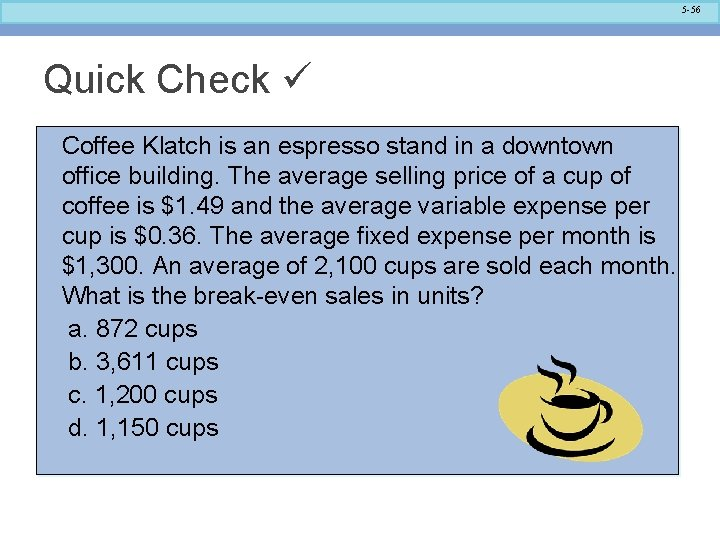5 -56 Quick Check Coffee Klatch is an espresso stand in a downtown office building. The average selling price of a cup of coffee is \$1. 49 and the average variable expense per cup is \$0. 36. The average fixed expense per month is \$1, 300. An average of 2, 100 cups are sold each month. What is the break-even sales in units? a. 872 cups b. 3, 611 cups c. 1, 200 cups d. 1, 150 cups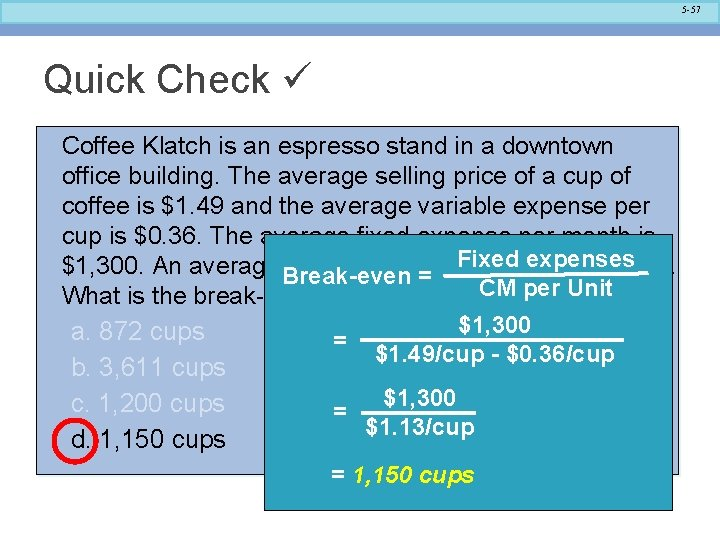5 -57 Quick Check Coffee Klatch is an espresso stand in a downtown office building. The average selling price of a cup of coffee is \$1. 49 and the average variable expense per cup is \$0. 36. The average fixed expense per month is Fixed expenses \$1, 300. An average Break-even of 2, 100 cups are sold each month. = What is the break-even sales in units? CM per Unit \$1, 300 a. 872 cups = \$1. 49/cup - \$0. 36/cup b. 3, 611 cups \$1, 300 c. 1, 200 cups = \$1. 13/cup d. 1, 150 cups = 1, 150 cups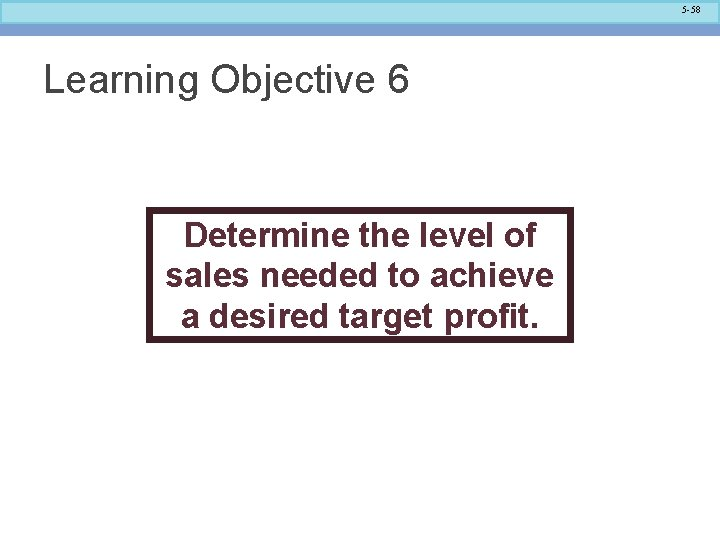5 -58 Learning Objective 6 Determine the level of sales needed to achieve a desired target profit.5 -59 Target Profit Analysis We can compute the number of units that must be sold to attain a target profit using either: (1) Equation method, or (2) Formula method.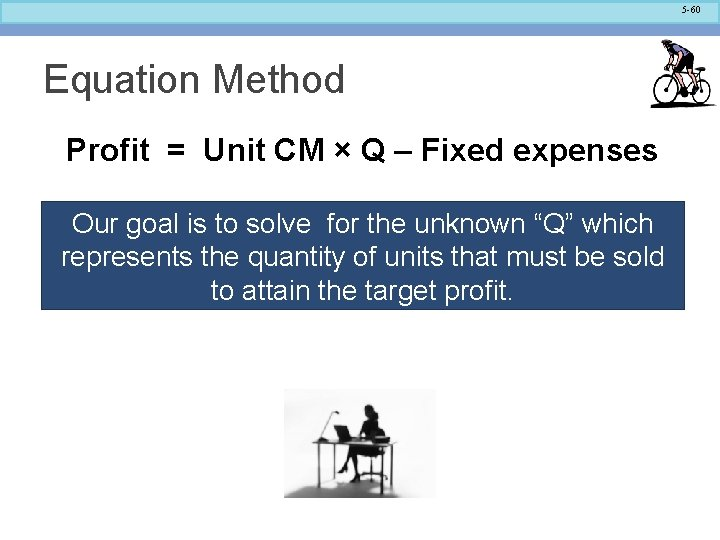5 -60 Equation Method Profit = Unit CM × Q – Fixed expenses Our goal is to solve for the unknown “Q” which represents the quantity of units that must be sold to attain the target profit.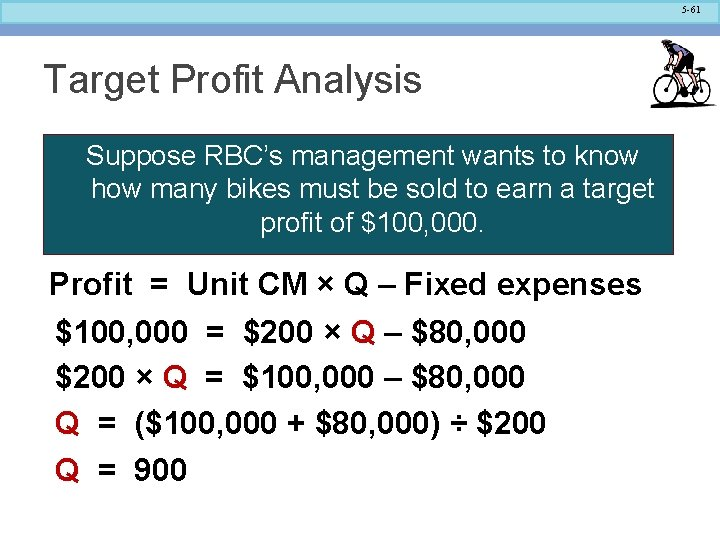5 -61 Target Profit Analysis Suppose RBC’s management wants to know how many bikes must be sold to earn a target profit of \$100, 000. Profit = Unit CM × Q – Fixed expenses \$100, 000 = \$200 × Q – \$80, 000 \$200 × Q = \$100, 000 – \$80, 000 Q = (\$100, 000 + \$80, 000) ÷ \$200 Q = 900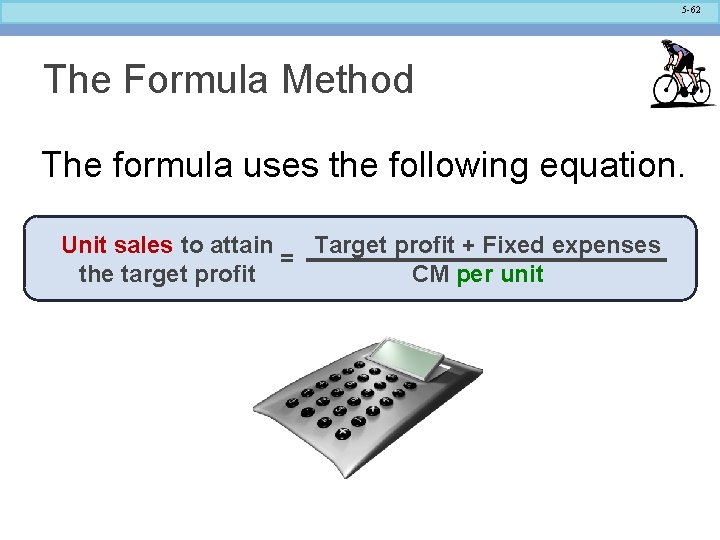5 -62 The Formula Method The formula uses the following equation. Unit sales to attain Target profit + Fixed expenses = the target profit CM per unit5 -63 Target Profit Analysis in Terms of Unit Sales Suppose Racing Bicycle Company wants to know how many bikes must be sold to earn a profit of \$100, 000. Unit sales to attain Target profit + Fixed expenses = the target profit CM per unit \$100, 000 + \$80, 000 Unit sales = \$200 Unit sales = 900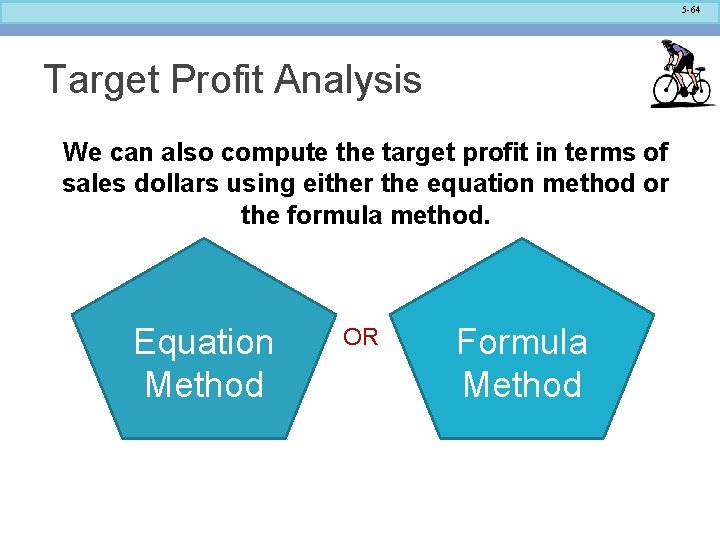5 -64 Target Profit Analysis We can also compute the target profit in terms of sales dollars using either the equation method or the formula method. Equation Method OR Formula Method5 -65 Equation Method Profit = CM ratio × Sales – Fixed expenses Our goal is to solve for the unknown “Sales, ” which represents the dollar amount of sales that must be sold to attain the target profit. Suppose RBC management wants to know the sales volume that must be generated to earn a target profit of \$100, 000 = 40% × Sales – \$80, 000 40% × Sales = \$100, 000 + \$80, 000 Sales = (\$100, 000 + \$80, 000) ÷ 40% Sales = \$450, 000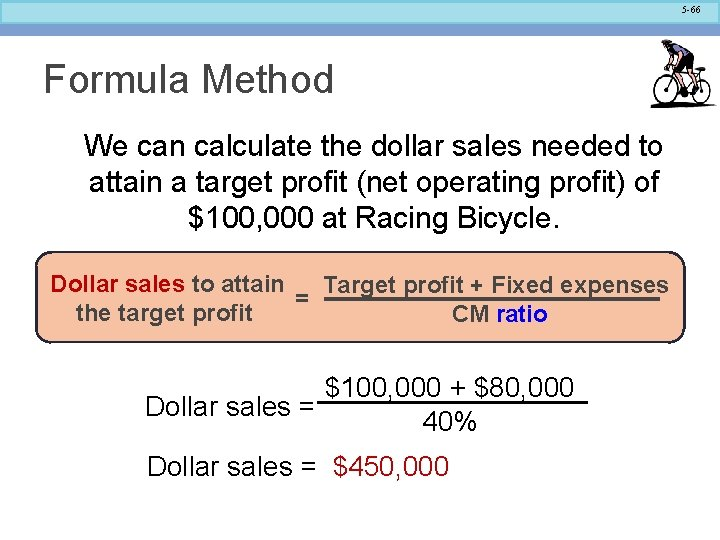5 -66 Formula Method We can calculate the dollar sales needed to attain a target profit (net operating profit) of \$100, 000 at Racing Bicycle. Dollar sales to attain Target profit + Fixed expenses = the target profit CM ratio \$100, 000 + \$80, 000 Dollar sales = 40% Dollar sales = \$450, 0005 -67 Quick Check Coffee Klatch is an espresso stand in a downtown office building. The average selling price of a cup of coffee is \$1. 49 and the average variable expense per cup is \$0. 36. The average fixed expense per month is \$1, 300. Use the formula method to determine how many cups of coffee would have to be sold to attain target profits of \$2, 500 per month. a. 3, 363 cups b. 2, 212 cups c. 1, 150 cups d. 4, 200 cups5 -68 Quick Check Coffee Klatch is an espresso stand in a downtown office building. The average selling price of a cup of coffee is \$1. 49 and the average variable expense per cup is salesfixed expense per month is \$1, 300. \$0. 36. The Unit average Target profit + Fixed expenses to attain = to determine. Unit Use the formula method how. CM many cups of target profit coffee would have to be sold to attain target profits of \$2, 500 + \$1, 300 \$2, 500 per month. = \$1. 49 - \$0. 36 a. 3, 363 cups b. 2, 212 cups \$3, 800 = \$1. 13 c. 1, 150 cups d. 4, 200 cups = 3, 363 cups5 -69 Quick Check Coffee Klatch is an espresso stand in a downtown office building. The average selling price of a cup of coffee is \$1. 49 and the average variable expense per cup is \$0. 36. The average fixed expense per month is \$1, 300. Use the formula method to determine the sales dollars that must be generated to attain target profits of \$2, 500 per month. a. \$2, 550 b. \$5, 013 c. \$8, 458 d. \$10, 5555 -70 Quick Check Coffee Klatch is an espresso stand in a downtown office building. The average selling price of a cup of coffee is \$1. 49 and the average variable expense per cup is \$0. 36. The average fixed expense per month is \$1, 300. Use the formula method to determine the sales dollars that must be generated to attain target profits of \$2, 500 per month. a. \$2, 550 b. \$5, 013 c. \$8, 458 d. \$10, 5555 -71 Learning Objective 7 Compute the margin of safety and explain its significance.5 -72 The Margin of Safety in Dollars The margin of safety in dollars is the excess of budgeted (or actual) sales over the break-even volume of sales. Margin of safety in dollars = Total sales - Break-even sales Let’s look at Racing Bicycle Company and determine the margin of safety.5 -73 The Margin of Safety in Dollars If we assume that RBC has actual sales of \$250, 000, given that we have already determined the break-even sales to be \$200, 000, the margin of safety is \$50, 000 as shown.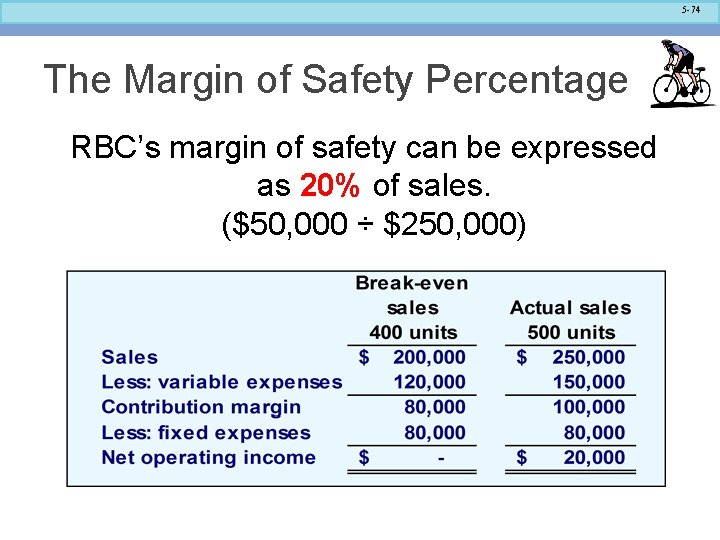5 -74 The Margin of Safety Percentage RBC’s margin of safety can be expressed as 20% of sales. (\$50, 000 ÷ \$250, 000)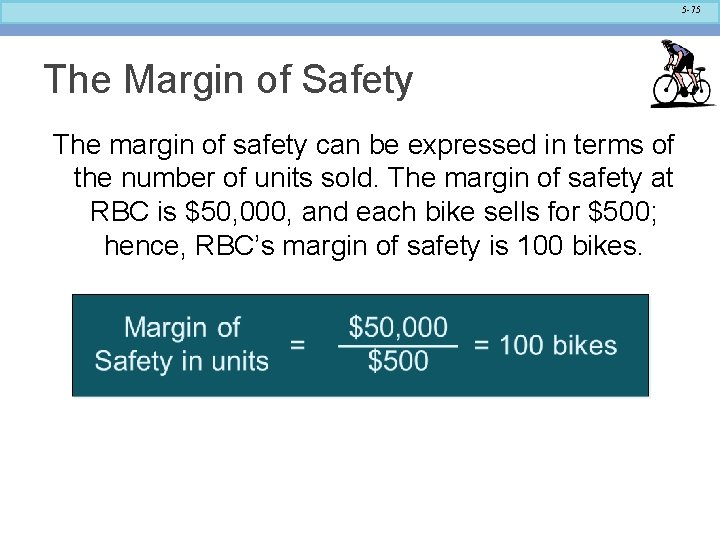5 -75 The Margin of Safety The margin of safety can be expressed in terms of the number of units sold. The margin of safety at RBC is \$50, 000, and each bike sells for \$500; hence, RBC’s margin of safety is 100 bikes.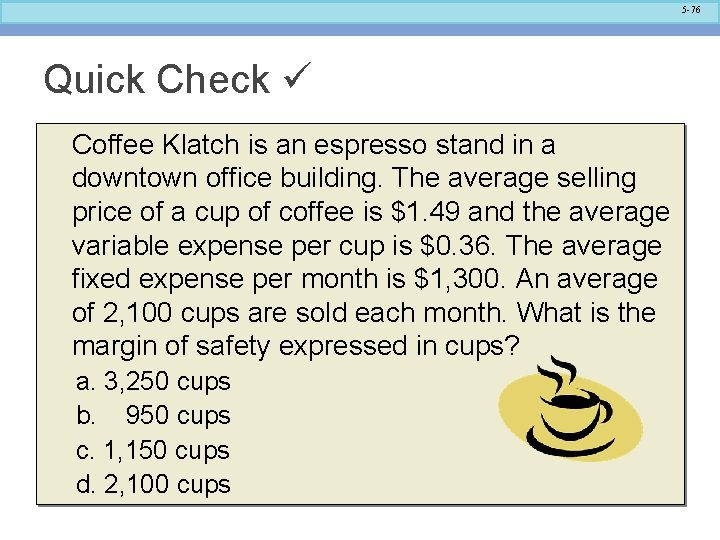5 -76 Quick Check Coffee Klatch is an espresso stand in a downtown office building. The average selling price of a cup of coffee is \$1. 49 and the average variable expense per cup is \$0. 36. The average fixed expense per month is \$1, 300. An average of 2, 100 cups are sold each month. What is the margin of safety expressed in cups? a. 3, 250 cups b. 950 cups c. 1, 150 cups d. 2, 100 cups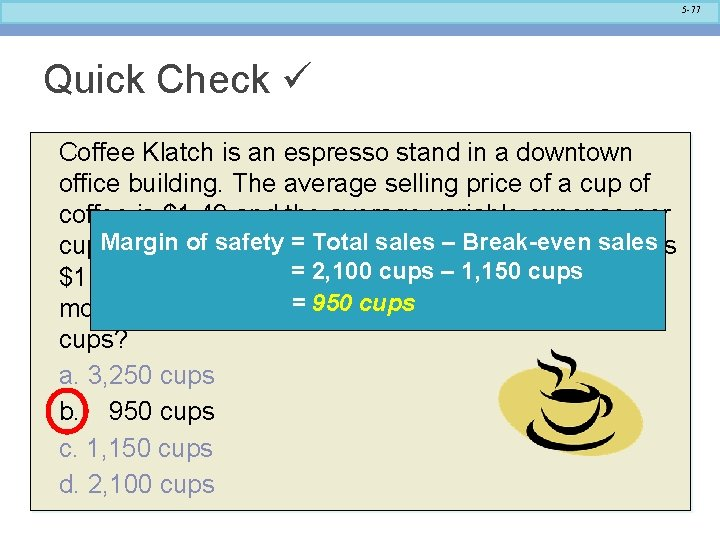5 -77 Quick Check Coffee Klatch is an espresso stand in a downtown office building. The average selling price of a cup of coffee is \$1. 49 and the average variable expense per of The safety = Totalfixed salesexpense – Break-even salesis cup. Margin is \$0. 36. average per month 1, 150 cups \$1, 300. An average =of 2, 100 cups– are sold each = 950 cups month. What is the margin of safety expressed in cups? a. 3, 250 cups b. 950 cups c. 1, 150 cups d. 2, 100 cups5 -78 Cost Structure and Profit Stability Cost structure refers to the relative proportion of fixed and variable costs in an organization. Managers often have some latitude in determining their organization’s cost structure.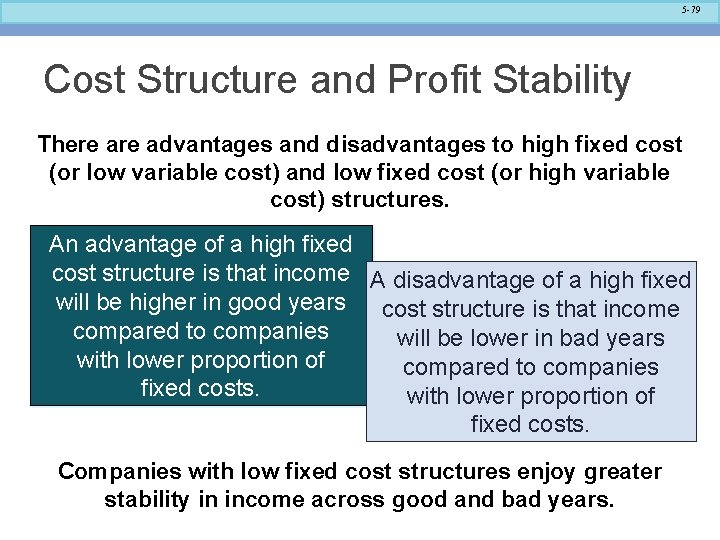5 -79 Cost Structure and Profit Stability There advantages and disadvantages to high fixed cost (or low variable cost) and low fixed cost (or high variable cost) structures. An advantage of a high fixed cost structure is that income A disadvantage of a high fixed will be higher in good years cost structure is that income compared to companies will be lower in bad years with lower proportion of compared to companies fixed costs. with lower proportion of fixed costs. Companies with low fixed cost structures enjoy greater stability in income across good and bad years.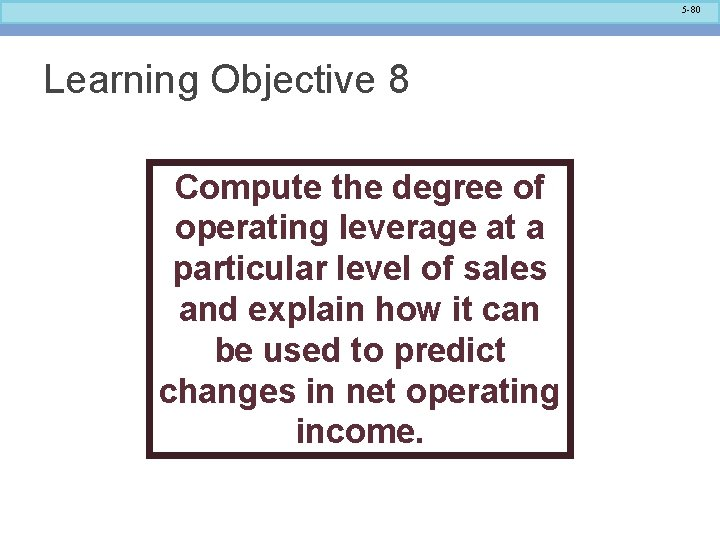5 -80 Learning Objective 8 Compute the degree of operating leverage at a particular level of sales and explain how it can be used to predict changes in net operating income.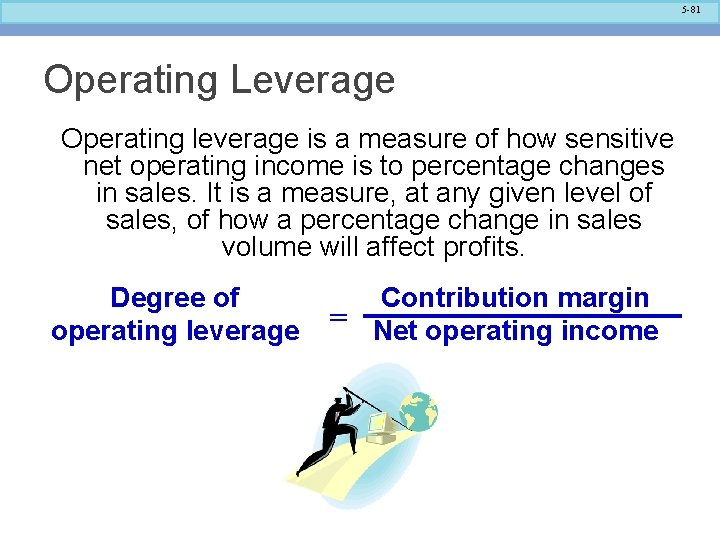5 -81 Operating Leverage Operating leverage is a measure of how sensitive net operating income is to percentage changes in sales. It is a measure, at any given level of sales, of how a percentage change in sales volume will affect profits. Degree of operating leverage Contribution margin = Net operating income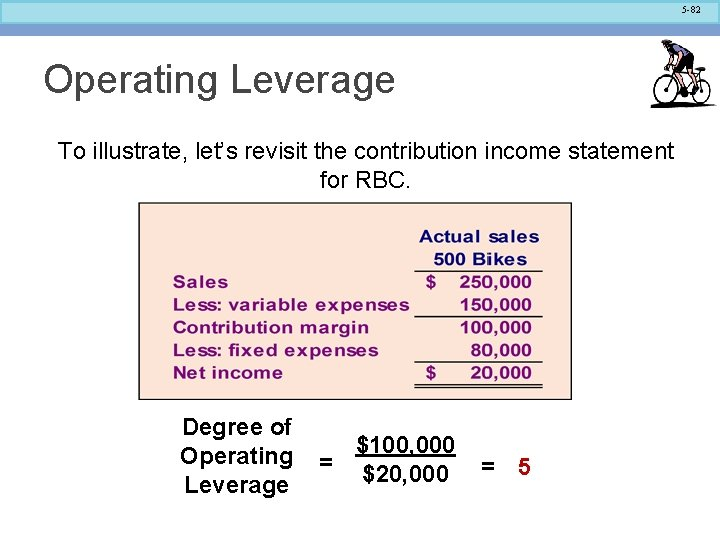5 -82 Operating Leverage To illustrate, let’s revisit the contribution income statement for RBC. Degree of Operating Leverage \$100, 000 = \$20, 000 = 55 -83 Operating Leverage With an operating leverage of 5, if RBC increases its sales by 10%, net operating income would increase by 50%. Here’s the verification!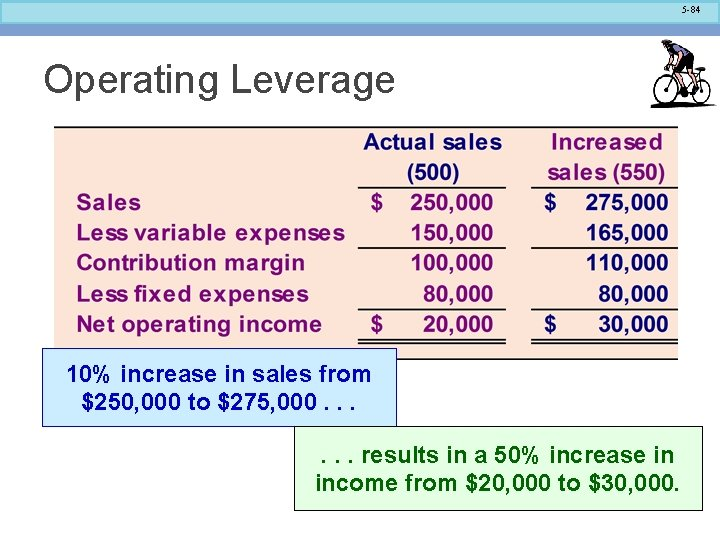5 -84 Operating Leverage 10% increase in sales from \$250, 000 to \$275, 000. . . results in a 50% increase in income from \$20, 000 to \$30, 000.5 -85 Quick Check Coffee Klatch is an espresso stand in a downtown office building. The average selling price of a cup of coffee is \$1. 49 and the average variable expense per cup is \$0. 36. The average fixed expense per month is \$1, 300. An average of 2, 100 cups are sold each month. What is the operating leverage? a. 2. 21 b. 0. 45 c. 0. 34 d. 2. 92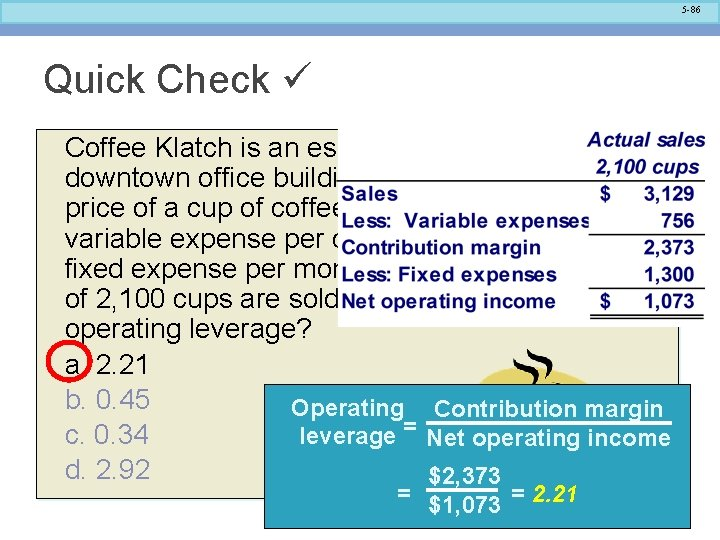5 -86 Quick Check Coffee Klatch is an espresso stand in a downtown office building. The average selling price of a cup of coffee is \$1. 49 and the average variable expense per cup is \$0. 36. The average fixed expense per month is \$1, 300. An average of 2, 100 cups are sold each month. What is the operating leverage? a. 2. 21 b. 0. 45 Operating Contribution margin c. 0. 34 leverage = Net operating income d. 2. 92 \$2, 373 = \$1, 073 = 2. 21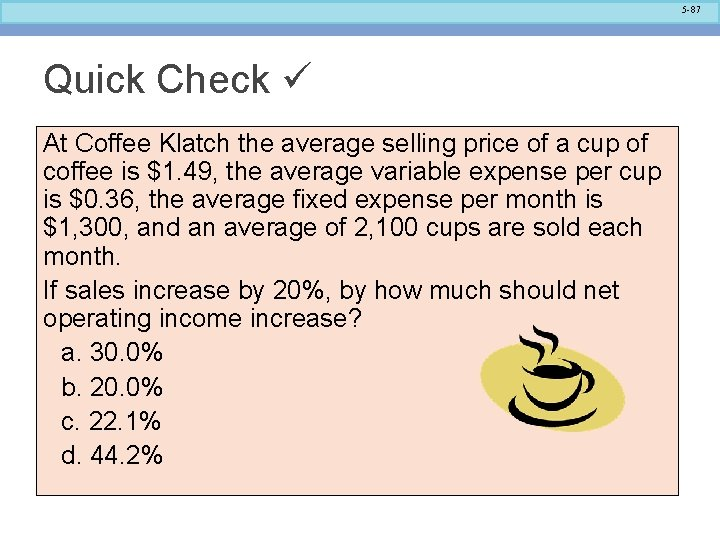5 -87 Quick Check At Coffee Klatch the average selling price of a cup of coffee is \$1. 49, the average variable expense per cup is \$0. 36, the average fixed expense per month is \$1, 300, and an average of 2, 100 cups are sold each month. If sales increase by 20%, by how much should net operating income increase? a. 30. 0% b. 20. 0% c. 22. 1% d. 44. 2%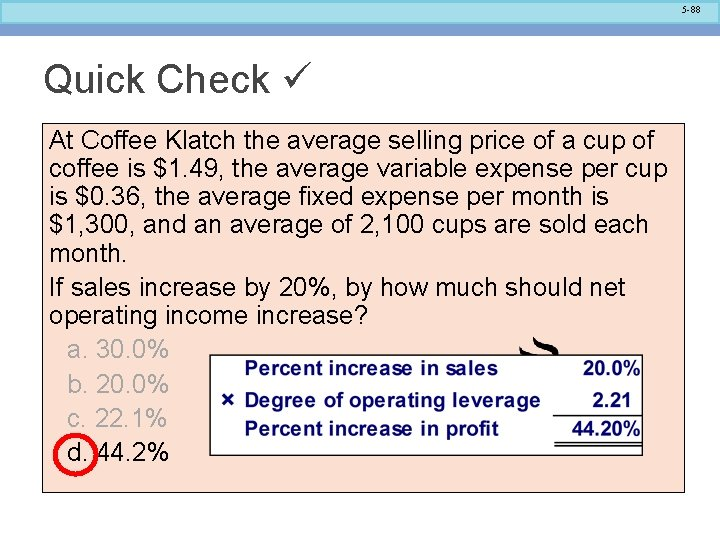5 -88 Quick Check At Coffee Klatch the average selling price of a cup of coffee is \$1. 49, the average variable expense per cup is \$0. 36, the average fixed expense per month is \$1, 300, and an average of 2, 100 cups are sold each month. If sales increase by 20%, by how much should net operating income increase? a. 30. 0% b. 20. 0% c. 22. 1% d. 44. 2%5 -89 Verify Increase in Profit5 -90 Structuring Sales Commissions Companies generally compensate salespeople by paying them either a commission based on sales or a salary plus a sales commission. Commissions based on sales dollars can lead to lower profits in a company. Let’s look at an example.5 -91 Structuring Sales Commissions Pipeline Unlimited produces two types of surfboards, the XR 7 and the Turbo. The XR 7 sells for \$100 and generates a contribution margin per unit of \$25. The Turbo sells for \$150 and earns a contribution margin per unit of \$18. The sales force at Pipeline Unlimited is compensated based on sales commissions.5 -92 Structuring Sales Commissions If you were on the sales force at Pipeline, you would push hard to sell the Turbo even though the XR 7 earns a higher contribution margin per unit. To eliminate this type of conflict, commissions can be based on contribution margin rather than on selling price alone.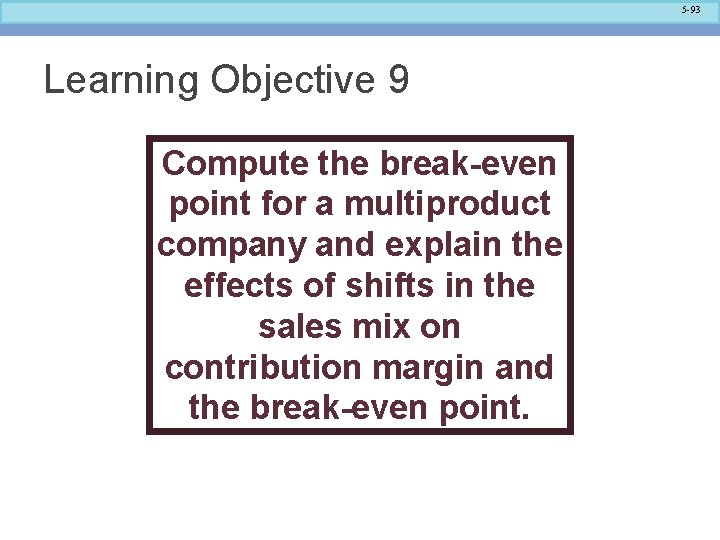5 -93 Learning Objective 9 Compute the break-even point for a multiproduct company and explain the effects of shifts in the sales mix on contribution margin and the break-even point.5 -94 The Concept of Sales Mix • Sales mix is the relative proportion in which a company’s products are sold. • Different products have different selling prices, cost structures, and contribution margins. • When a company sells more than one product, break-even analysis becomes more complex as the following example illustrates. Let’s assume Racing Bicycle Company sells bikes and carts and that the sales mix between the two products remains the same.5 -95 Multi-Product Break-Even Analysis Bikes comprise 45% of RBC’s total sales revenue and the carts comprise the remaining 55%. RBC provides the following information: \$265, 000 = 48. 2% (rounded) \$550, 000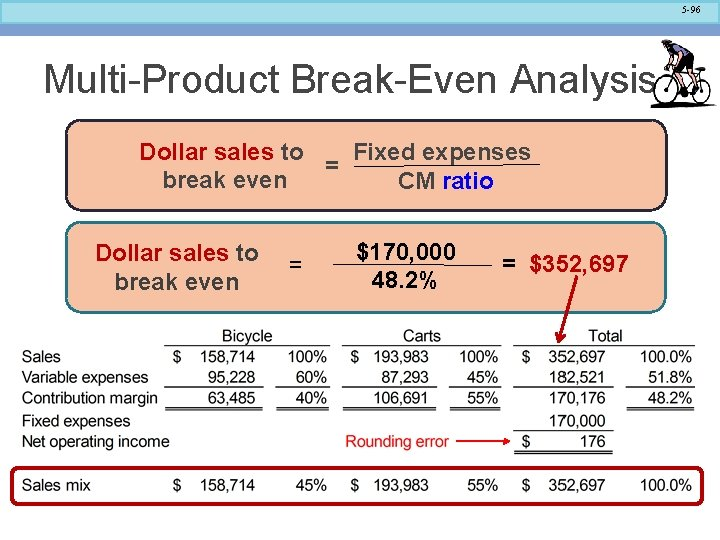5 -96 Multi-Product Break-Even Analysis Dollar sales to Fixed expenses = break even CM ratio Dollar sales to break even = \$170, 000 48. 2% = \$352, 6975 -97 End of Chapter 5